The dynamic marginal tax rate

Thomas Cool

Scheveningen, Holland

cool@can.nl, http://www.can.nl/~cool

August 26 1997

JEL A, H2, H3, J2, J3, J5

Summary

There is a general misconception on the impact of marginal tax rates. Following the hypothesis of optimising economic agents, it is proper that marginal rates enter the discussion. The only issue is that the margin must be computed correctly. In dynamics, one must take account of the fact that tax parameters may change, or for optimality should change. The change of tax parameters affects the value of the proper marginal rate. The proper dynamic marginal rate might be called the ‘dynamic marginal rate’. The revolution in dynamics as exemplified by Keynes (1936) may find another twist in this issue.

This new result has dramatic consequences for common perceptions in macro economic policy. It is commonly thought that stagflation has been caused by external factors such as technology or the competition of low wage countries. The current analysis points to the conclusion that stagflation has been caused by internal factors, notably government policy itself, which policy has been based on a wrong perception of the marginal tax rate.

Introduction

Most developed nations suffer from an unfavorable combination of inflation and unemployment, sometimes associated with stagnation in economic growth (stagflation). Taxes commonly show up in the analysis due to their possible effect on labour supply, work effort and forward shifting of the tax burden.

Since stagflation is a common feature in developed nations, there is some ground to look at the common features in taxes. Most developed nations have nonproportional taxes, i.e. tax codes with an exemption at the threshold and then a (rising) statutory marginal rate. The latter parameters in fact concern the intercept and the slope of the tax function. There is also a remarkable similarity in the policy regarding these two parameters, see OECD (1986):

• The policy concerning the intercept or exemption.
Exemption generally is low, also with respect to social insurance.
Tax parameters, and notably exemption, are generally indexed on inflation. Since incomes tend to grow faster than inflation, exemption lags behind incomes. There is a deliberate tax creep - measured by the ‘macroeconomic progression factor’.
• The policy concerning the slope or the statutory marginal rate.
Both in theory and public discussion there is a consideration that high marginal rates have disincentive effects. This has resulted in the policy objective to reduce marginal rates. One way to reduce marginal rates has been the switch from income tax to VAT.

Given the common notion of budget neutrality, these two feature in policy tend to complement each other. Budget neutrality requires that the revenue loss due to slope reduction is compensated for by other proceeds. These other proceeds will often come from the tax creep and the reduction of exemption. At least, it is often thought that the reduction of exemption generates additional revenue.

Above lines summarise the currently conventional view. Cool (1992a, 1994a) reacts to this conventional view and policy in the following manner:

1. If exemption is lower than subsistence, then a higher minimum wage is required, causing unemployment (so less revenue and more outlays). The latter seems to be the general situation in the OECD. A prime cause is the differential indexation, i.e. that exemption is indexed on inflation while subsistence rises with the general growth of welfare.
2. The economic literature shows a conceptual problem, or paradox, on marginal rates. Statutory marginal rates are important in popular understanding, but not in the empirical data.
1. Research, as witnessed by the existing literature such as Gelauff (1992), deals better with the data, but doesn’t convince the popular view.
2. There is my new analysis regarding the dynamic marginal tax rate.

Recently, the Dutch Central Planning Bureau (1997) discussed some aspects of my analysis. The occasion is that the political party De Groenen (‘The Greens’) - of which I am not a member - has taken interest in that analysis, and, with the elections of 1998 coming up, that party asked the CPB to look into some scenarios. Unfortunately, the CPB report shows a misunderstanding on some key elements. So it seems useful to discuss some points at greater length, which is what we shall be doing here.

Our analysis starts with a nonlinear tax function that is a useful tool for discussing the analysis on the dynamic marginal tax rate.

Nonlinear tax function

We shall use T[y] to denote a general tax function on income y. We shall also use two special tax functions. First, the Bentham tax function:

Bentham[y] = r (y - x) (y > x)

with exemption x and marginal rate r. This function is linear but already results into nonproportional taxes. Governments in practice have nonlinear tax schemes that give stronger nonproportionality, reflecting political views on the redistribution of income.

Strong nonproportionality has a special effect. Since taxes in the 1960s were more nonproportional than nowadays, the tax structure combined with the lognormal shape of the employment function, and generated strong nonlinear effects and a strong upswing of the NAIRU in the early phase of stagflation.

For this reason it is useful to introduce a flexible tax function with one more parameter than Bentham’s function, i.e. to incorporate some curvature. The use of this new function allows us to give concrete examples whenever nonlinearity is useful. For clarity, it appears that this function can approximate the actual Dutch tax situation. The tax function is: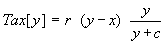(y > x)

with y the tax base and x the exemption or threshold, r the marginal rate in the limit when y goes to infinity, and c a curvature parameter. The ordered set of parameters is q = (r, x, c). We do not use Greek symbols for these parameters since we will regard them as key strategic variables. If governments would use this function for practical tax collection, they might note (1) that exemption would be determined by subsistence, (2) that r would follow from the limit marginal rate for the highest incomes, (3) so that curvature c would follow from required total revenue and the income distribution. Use of this function thus both allows for a decent degree of nonproportionality and would reduce much of political debate about positioning of tax brackets and rates.

The average tax is:The marginal rate on the marginal dollar can always be approximated as Tax[y + \$1] - Tax[y] so that the common tax payer will have no problem in determining it. The proper formula itself however is not too simple. At y = x it starts with the value r x / (c + x) and in the limit it equals r. For the whole range:(T.1)

Note that the tax function can be transformed into a linear format consisting of income, average tax and a constant:

Tax[y] = r.y - r.x - c.Tax[y] / y = a1.y + a2 + a3.(Tax[y] / y)

Cool (1992) used this relation for a simple linear least square estimation that neglects the error on the average on the right hand side, using 1988 Dutch data for 12 selected income levels. The result was:(in 1988 \$ thousand)

The equation can be plotted for two ranges, (H1) for a low income range till \$25 thousand to show the curvature, and (H2) for a wider income range till \$250 thousand to show the straightness in the limit. In a plot, the 45-degree line is usefully added to allow visualisation of net income. Since the Dutch estimate has a high marginal rate in the limit of 57.2 %, we add US-alike lines (U1) and (U2) with a r = 40 % limit. The two ranges are plotted in figure a.

Figure A: Different tax regimes
(H) Holland, (U) US-alikeExemption

Though the present analysis is basically about the dynamic marginal, it is useful to recall some aspects of Cool (1992a, 1994a) in order that one better understands what the discussion is about.

The nonproportional tax clearly becomes important when incomes differ, i.e. labour is heterogeneous in terms of productivity, labour costs and income. Lower income earners are affected disproportionally by the exemption level, not merely in terms of the income distribution but also in terms of their competitive position versus higher earners.

The minimum wage M consists of two elements, namely net subsistence B and the tax on M. For above tax function: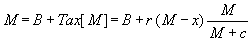This can be solved as M[B, r, x, c]. An easy solution is M = B when x = B. However, with differential indexation of B and x,notably B in income and x on inflation only, M grows faster than productivity too, causing unemployment. Cool (1992a, 1994a) provides the proofs here.

figure b summarises the situation for a linear function, with c = 0. figure b has been constructed as follows:

1. The line T(y) gives the tax function, with exemption x. The current Dutch tax regime has an exemption of Dfl 7 thousand and first bracket of about 40%, while premiums raise the marginal rate to above 50%.
2. Also drawn is the 45° line. The difference between the tax line and the 45° line gives net income.
3. The line y - B parallel to the 45° line gives income net of subsistence. In other words, the subsistence line secures part of net income that is needed to subsist. In Holland B = 21.
4. The minimum wage M can be found at the intersection of T[y] and y - B, for Holland at M = 35.
5. We then draw a lognormal employment distribution, which gives the number of people (manhours) at the various levels of income (productivity). The employment distribution is dotted below the minimum wage, since it is not observed because those workers are unemployed. One can see that the tax code below the minimum wage has no real meaning, while it drives up the minimum wage.

Figure B: Taxes, employment and minimum wage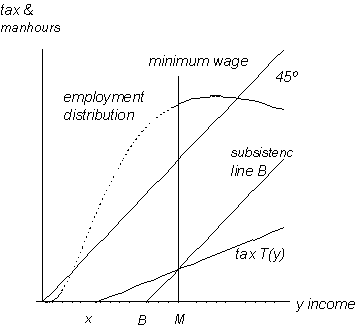This analysis points to the suggestion of "waiving taxes for the lowly productive" which can be translated as "raising exemption" so that x = B. Interestingly, this suggestion appears to provoke some terminological confusions. The notion of "raising exemption" is often taken to imply that all other brackets shift along with exemption. This causes a huge loss of tax revenue. E.g. Gelauff (1992), who uses the official general equilibrium model of the Central Planning Bureau to compute the economic impact of raising exemption, adopts this expensive approach.

However, there are some alternative implementations. Their common feature is:

• Taxes above the current minimum wage need not be changed, since those taxes are not the problem.
• When changing taxes up to minimum wage M, be aware that there will be no revenue loss below M, since people may not work below the legal minimum wage, and thus don’t earn and don’t pay taxes.
• The old net minimum (subsistence) becomes the new gross minimum. Of course, people currently earning the minimum wage keep earning the old gross minimum, so are not affected adversely.

There are two extreme forms to raise exemption costlessly:

1. Introduce a new separate ‘tax group’ that only holds for workers below the current minimum wage. This group has exemption at the old net minimum wage and a marginal rate of say 50%. Clearly, there is jump in taxes at the current minimum wage. However, the high exemption can be said to apply to all citizens, though many do not fall in the new group so that they do not quality. (The latter is only unfortunate for them, if they prefer a high exemption above their current high income.)
2. One might opt for a 100% marginal rate between subsistence and the current gross minimum wage. In this case there is no tax jump. High exemption again applies to all citizens, but its effect is undone by the high marginal rate region. A 100% marginal rate need not be considered to be a bad situation, for the following two reasons:
1. The 100% rate can be reduced in the near future, using national income growth. Hence, net income positions in the 100% range will not differ in the beginning, but will start to differ soon. Hence workers will have an incentive to ask for wages that fit their level of productivity.
2. See the analysis on the marginal tax rate, below.

These two extremes, and all possibilities between them, warrant the conclusion that current minimum wage unemployment is inefficient. There exists a Pareto improvement, costless, where some can advance without anybody losing anything.

More practical solution methods

It appears that above two extreme positions are hard to communicate, even to trained economists. People expect solutions to costs something, and they are more likely to accept a solution method to unemployment that involves costs. It may be that more practical solutions will affect the above-M region where costs are involved.

However, solutions that affect the above-M region may still be net-zero solutions. When employment is generated, the state saves on benefit payments, and some revenue can be used to reduce taxes above the current minimum wage.

A first solution method uses a linear line, as is given by figure c. For Holland, it uses exemption of 21, a 75% percent rate till about 50, and then continues on the existing tax line. The section where costs are involved is shaded.

Figure C: Linear repairAn alternative is a nonlinear trajectory from subsistence to some place in the current regime. figure d gives an example of such nonlinear trajectory, where the function Tax[.] has been estimated to fit the 1997 Dutch tax code (inclusive of premiums) but with a nonlinear repair towards subsistence. The special point is that this estimated Tax[.] has a negative curvature parameter.

Figure D: Nonlinear repairThe curved alternative starts at B and joins T[y] at a point approx. y = 50. The only revenue loss will occur in section from M to approx. y = 50.

We will discuss the optimal regime elsewhere. These few pages were useful to clarify some terminological confusions. It also indicates that marginal rates will feature strongly in the discussion about the repair. A marginal rate of 100% or ‘only’ 75% or the marginal rates associated with negative curvature indeed do need some explanation.

The marginal rate

As said, economic literature shows a conceptual problem, or paradox, on marginal rates. Statutory marginal rates are important in popular understanding, but not in the empirical data. Research, as witnessed by the existing literature such as Gelauff (1992), deals better with the data, but doesn’t convince the popular view. The following analysis suggests a solution.

Partial versus total derivative

Conventional theory, public discussion and empirical research generally use statutory rates as the "marginals". With T[y] the tax associated with income y, the marginal rate commonly is computed as T[y]/ y. For our function this is the partial derivative as used in equation (T.1). However, the tax function is better understood not as T[y] but as the multivariate T[y, q] with q the (now arbitrary) tax parameters. Agents will tend to take account of parameter changes. So optimisation remains our paradigm - and it results into marginal rates - but the better marginal rate is the total derivative, or dynamic marginal rate (DMR):

dT[y, q] T[y, q] T[y, q]
----------- = ------------ + ------------ dq / dy
dy y q

The topic of discussion is dq / dy. To proceed from this point, it appears didactically useful to first restate the common reaction to the DMR, and then develop the new analysis.

A conventional reaction

The conventional reaction is that tax parameters may be indexed to national income Y, but are not indexed to personal income. The individual agent in the economy will not think that his change in income can affect national tax parameters. Hence dq / dy should be zero.

Let us use the Bentham tax function for ease of exposition. Let us assume that only exemption is indexed on national income, and in continuous form the indexation reads as x = x Y with x as a fixed value for a base year. Thus:

T[y] = Bentham[y, x Y] = r (y - x Y)

It appears that x is very small. For example, with E the number of tax payers (employment), and Y / E average income, we may take exemption as a third of average income, so that x = x / Y = 1 / (3 E) which thus is very small. But the small size does not invalidate the indexation method, since:

dLog[x] = dLog[x Y ] = dLog[Y]

Note that Y is the sum of all incomes. An income change for an individual does not affect the income changes of others. Assuming that other incomes stay fixed, we find for an individual income dY / dy = 1. If y rises and no other income rises, then the growth of national income dLog[Y] is equal to the growth for the single person weighted by its share in total income:

dLog[Y] = (y / Y) dLog[y]

It follows that the marginal tax for the individual is:

d T[y] / dy = r (1 - x )

Now, since x is such a small number, the marginal rate is virtually equal to r.

In general we find:

dq / dy = (dq / dY) . ( dY / dy) = dq / dY

Since dY / dy = 1. If parameters are indexed on national income, then dLog[q] = dLog[Y] and then dq / dY = q / Y so that

dq / dy = q / Y

which is close to zero since parameters q are generally much smaller than national income. We conclude that dq / dy is not quite zero, but practically zero, and this seems to corroborate the conventional reaction to the DMR.

Hence the conventional reaction to the DMR is that the DMR does not change the traditional analysis on marginal rates. Hence there is no hope for unemploment along these lines. With ongoing technological growth and competition of low wage countries, only the flexibility of labour markets will help to reduce unemployment, even if this means a reduction of net minimum wages. That, at least, is the conventional reaction.

The expectations revolution

However, Keynes (1936) explained that proper dynamic analysis inherently means that we have to consider expectations.

In this case the agent will be aware that parameters are indexed in some manner. Due to indexation, the term dq / dy can take significant values. Let q be indexed on national income growth Y. For many tax functions the indexation of parameters may take the form dLog[q] = dLog[Y], as can be done for exemption and curvature of Tax[y]. If dLog[q] = dLog[Y] thenThis again may reduce to the q / Y above. However, if we now take expectations of the growth of national income, which means that the agent assumes that the other incomes do not remain constant, then: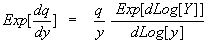Thus, next to knowledge about indexation, the agent will have expectations about the national income growth dLog[Y], and compare his own growth of income dLog[y] to this expectation. In terms of expectations, dq /dy does not vanish to zero. This is especially relevant when the parameter q gives exemption x that is a sizeable part of income.

So there is hope for the unemployed.

Discrete form

Above can also be formulated in discrete form. Indexation generally takes place with a lag, and then the discrete DMR is more adequate. This is:

DMR[y] = (T[y, q] - T[y-1 , q-1 ]) / (y - y-1 ) = D T / D y

When exemption follows national income, then the dynamic marginal is lower than the static marginal. The reason is that the rise of exemption benefits the net income position even before the personal income growth is taken into account.

In fact, when tax parameters are indexed such that the tax has the same growth rate as income, then the dynamic marginal rate equals the average tax rate. This holds for individuals and for the macro data if all individuals are on a balanced growth path. For nonzero values: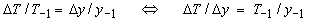The following is a small example of how a dynamic marginal rate can equal a normal average. Let exemption be \$10000, and let the statutory marginal rate thereafter be 50%. Someone earning \$50000 pays the tax of \$20000, on average 40%. Let all incomes grow 5%, and exemption be indexed on national income. Then exemption becomes \$10500, income \$52500, tax \$21000, again 40%. Thus on the (dynamic) "marginal dollar" this person doesn't pay 50% but 40%.

For the Bentham tax function we can derive a simple expression for individual growth. Let personal income grow by rate g, so that y[+1] = (1 + g) y, and let exemption be expected to be adjusted by rate h, so that x[+1] = (1 + h) x. Then we find: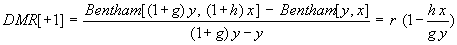Let us regard the dynamic marginal rate for a Dutchman in 1997 who considers an increase in work effort for 1998 (and beyond), and let us assume a regime of sound economics. In the ideal case, exemption in the base year is put at subsistence, in this case Dfl 21 thousand. Ideally, subsistence rises with income, and not just real net average incomes. This ideal implies that exemption is adjusted not just for inflation, but for the nominal growth of income. Let us assume this ideal, and let us assume that nominal growth is 4%, consisting of 2% inflation and 2% real growth. Let us then regard the situation of a single economic agent. He knows that next year exemption will be adjusted with 4%. He has to judge whether it is worthwhile to him to invest or to increase labour effort, so that his income will rise. If his income rises with 4%, then his dynamic marginal will be equal to his present average tax rate. If his income rises by 8%, then his dynamic marginal will differ; it will depend upon his actual income level, but anyway will be less than the statutory marginal rate of 50%. figure e gives the plot of the dynamic marginal for those two rates, for various levels of income

Figure E: The dynamic marginal rate

Individual income grows at 4% or 8%, while national income grows at 4%
and the statutory marginal rate is 50%For Tax[.] the rule is that parameters x and c are indexed on y. With the index for y as i = P ryi, we find for the (individual) average tax burden that the index drops from both numerator and denominator:

Tax[ i y; r, i x, i c] / (i y) = r (i y - i x) / (i c + i y) = Tax[y; r, x, c] / y ( i > 0)

The case of a balanced growth path is depicted in figure f. Let us take the example of a doubling of income. Point A is an arbitrary point on the employment density. We scale the density so that A also lies on the tax function (H). For that arbitrary income at A we determine the average tax as a ray through A and the origin. Now, if all incomes double, then the employment frequency density shifts, and A becomes 2A. If tax parameters x and c double too, then the tax function becomes (2H). At 2A the individual pays tax C, which is the same average tax as in A.

Figure F: A balanced growth shift
A-2A: constant frequency, A-C: the same average taxHow do agents react when there is no balanced growth ? Indexation to national income can be said to be "neutral to the income change". The tax choices facing an individual, whose income grows as national income, are constant. The utility reaction thus depends on the change of income itself. It may be that an individual, whose income might grow as fast as national income, decides to grow differently, depending upon his leisure-income utility. Since the context is that all individuals are adjusting, this may be reformulated as that individuals are determining their place within the income distribution.

Our analysis thus suggests that tax incentives primarily affect decisions about one’s place in the income density. Any individual change that differs from the national average can be interpreted, or defined, as the individual decision to accept another place in the income distribution. It would be interesting to reinterprete economic models on growth in these terms, and see whether elegant regularities can be found or constructed. However, it leads too far to really look into this matter, since it is not our proper subject.

We conclude that indexation and expectations about the growth of national income (relevant for indexation) lead to different results than the conventional view on marginal rates.

Policy simulations

There is one area where the DMR cannot easily be overlooked. This is the area of policy simulation, where tax adjustment cannot be neglected. For sure, empirical analyses and government projections indeed deal with tax parameter changes. For example the well-known Reagan tax cuts were put into the forecasts at that time. However, the analysis above focusses our attention on the impact on individual behaviour, where we regard the marginal calculation by agents themselves.

Let us regard policy simulations using common practical economic models. Let us for example regard the effects of a rise of government investments as financed by taxes, for a sustained period of 8 years (two presidential terms). To do a simulation properly, the tax function used must reflect government policy, which includes indexation. For example, exemption and other brackets are adjusted for last year inflation while the statutory marginal rates remain the same. The different investment paths result in different paths for the taxes. This is not just a model result, but also the agents in the economy would encouter different regimes. Thus the model generates different dynamic marginal rates, while the agents are assumed to react only to the same rates. The situation gets even complexer when the alternative policy includes a different indexation scheme, such as indexation of taxes on national income. These certainly are situations where one can start wondering about dynamic consistency (not to be confused with the ‘dynamic consistency of policy’ as another topic in economic literature).

It might even be, then, that the best way to understand the dynamic marginal rate is to see it as a solution to this kind of dynamic inconsistency.

Conclusion

There is a general misconception on the impact of marginal tax rates. Following the hypothesis of optimising economic agents, it is proper that marginal rates enter the discussion. The only issue is that the margin must be computed correctly. In dynamics, one must take account of the fact that tax parameters may change, or for optimality should change. The change of tax parameters affects the value of the proper marginal rate. The proper dynamic marginal rate might be called the ‘dynamic marginal rate’. The revolution in dynamics as exemplified by Keynes (1936) may find another twist in this issue.

This new result has dramatic consequences for common perceptions in macro economic policy. It is commonly thought that stagflation has been caused by external factors such as technology or the competition of low wage countries. The current analysis points to the conclusion that stagflation has been caused by internal factors, notably government policy itself, which policy has been based on a wrong perception of the marginal tax rate.

References

Note: econwpa references are the locations of the papers on the Economics Working Papers Archive of the Washington University of St. Louis, Missouri, USA, Http://econwpa.wustl.edu

Aronson, E. (1972), "The social animal", Freeman, sixth edition 1992

Centraal Planbureau (1997), "Commentaar op enige beleidsvoorstellen van de Groenen", Notitie June 26

Cool, T. (1992a), "Definition and Reality in the general theory of political economy; Some background papers 1989-1992", Magnana Mu Publishing & Research (English & Dutch)

Cool, T. (1992b, 1995c), "On the political economy of employment in the welfare state", revised paper taken from (1992a) (econwpa mac/9509001)

Cool, T. (1994a, 1995a), "Tax structure, inflation and unemployment", Magnana Mu Publishing & Research (econwpa mac/9508002)

Cool, T. (1994b), "Trias Politica & Centraal Planbureau", Samuel van Houten Genootschap (Dutch)

Cool, T. (1995b), "How a dead wrong OECD tax policy causes mass unemployment: An explanation using data for Holland 1950-1995" (econwpa mac/9508003)

Cool, T. (1995d), "Belastingstructuur, inflatie en werkloosheid", paper presented for the "Nederlandse Arbeidsmarktdag 1995" (Dutch)

Cool, T. (1996a), "Unemployment solved ! A breakthrough in economic analysis", econwpa get/9604002

Gelauff (1992), "Taxation, social security and the labour market", Thesis Katholieke Universiteit Tilburg

Keynes (1936), "The general theory of employment, interest and money", MacMillan

OECD (1986), "An empirical analysis of changes in personal income taxes," Paris